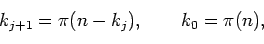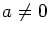Journal of Integer Sequences, Vol. 24 (2021), Article 21.10.5

## On the Solution of the Equation n = ak + bpk by Means of an Iterative Method

### Juan Luis Varona Departamento de Matemáticas y Computación Universidad de La Rioja Logroño Spain

Abstract:

For fixed positive integers n, we study the solution of the equation n = k + pk, where pk denotes the kth prime number, by means of the iterative methodwhich converges to the solution of the equation, if it exists. We also analyze the equation n = ak + bpk for fixed integer valuesand b>0, and its solution by means of a corresponding iterative method. The case a>0 is somewhat similar to the case a=b=1, while for a<0 the convergence and usefulness of the method are less satisfactory. The paper also includes a study of the dynamics of the iterative methods.

Full version:  pdf,    dvi,    ps,    latex

(Concerned with sequences A000045 A014688 A259180.)

Received March 21 2021; revised versions received March 23 2021; November 16 2021; November 21 2021. Published in Journal of Integer Sequences, November 27 2021.